GeeksforGeeks App
Open AppBrowser
Continue

# Append data to an empty Pandas DataFrame

Let us see how to append data to an empty Pandas DataFrame.

Creating the Data Frame and assigning the columns to it

 `# importing the module``import` `pandas as pd`` ` `# creating the DataFrame of int and float``a ``=` `[[``1``, ``1.2``], [``2``, ``1.4``], [``3``, ``1.5``], [``4``, ``1.8``]]``t ``=` `pd.DataFrame(a, columns ``=``[``"A"``, ``"B"``])`` ` `# displaying the DataFrame``print``(t)``print``(t.dtypes)`

Output :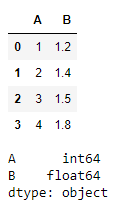On appending the float values to the int valued data type column  the resultant data frame column type-caste into float in order to accommodate the float value

If we use the argument `ignore_index = True`  => that the index values will remain continuous instead of starting again from 0, be default it’s value is `False`

 `# Appending a Data Frame of float and int``s ``=` `pd.DataFrame([[``1.3``, ``9``]], columns ``=` `[``"A"``, ``"B"``])``display(s)`` ` `# makes index continuous``t ``=` `t.append(s, ignore_index ``=` `True``)  ``display(t)`` ` `# Resultant data frame is of type float and float``display(t.dtypes)  `

Output :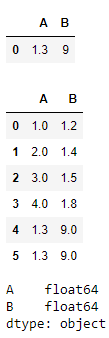When we appended the boolean format data into the data frame that was already of the type of float columns then it will change the values accordingly in order to accommodate the boolean values in the float data type domain only.

 `# Appending a Data Frame of bool and bool``u ``=` `pd.DataFrame([[``True``, ``False``]], columns ``=``[``"A"``, ``"B"``])``display(u)``display(u.dtypes)`` ` `t ``=` `t.append(u)``display(t)``display(t.dtypes)  ``# type casted into float and float`

Output :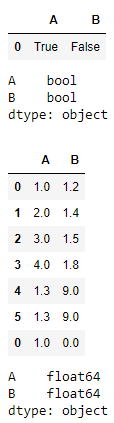On appending the data of different data types to the previously formed Data Frame then the resultant Data Frame columns type will always be of the wider spectrum data type.

 `# Appending a Data Frame of object and object``x ``=` `pd.DataFrame([[``"1.3"``, ``"9.2"``]], columns ``=` `[``"A"``, ``"B"``])``display(x)``display(x.dtypes)`` ` `t ``=` `t.append(x)``display(t)``display(t.dtypes)`

Output :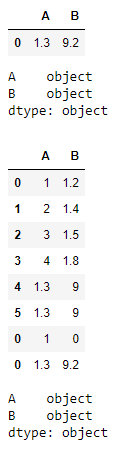If we aim to create a data frame through a for loop then the most efficient way of doing that is as follows :

 `# Creating a DataFrame using a for loop in efficient manner``y ``=` `pd.concat([pd.DataFrame([[i, i ``*` `10``]], columns ``=` `[``"A"``, ``"B"``])``               ``for` `i ``in` `range``(``7``, ``10``)], ignore_index ``=` `True``)`` ` `# makes index continuous``t ``=` `t.append(y, ignore_index ``=` `True``)  ``display(t)``display(t.dtypes)`

Output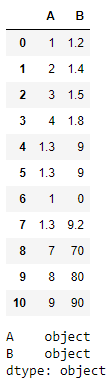If we attempt to add different column than already in the data frame then results are as follows :

 `# Appending Different Columns``z ``=` `pd.DataFrame([[``"1.3"``, ``"9.2"``]], columns ``=` `[``"E"``, ``"F"``])``t ``=` `t.append(z)``print``(t)``print``(t.dtypes)``print``()`

Output :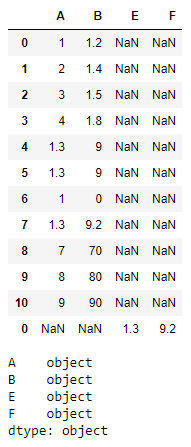My Personal Notes arrow_drop_up